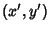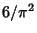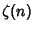## Visible PointTwo Lattice Pointsandare mutually visible if the line segment joining them contains no further Lattice Points. This corresponds to the requirement that, wheredenotes the Greatest Common Divisor. The plots above show the first few points visible from the Origin.

If a Lattice Point is selected at random in 2-D, the probability that it is visible from the origin is. This is also the probability that two Integers picked at random are Relatively Prime. If a Lattice Point is picked at random in-D, the probability that it is visible from the Origin is, whereis the Riemann Zeta Function.

An invisible figure is a Polygon all of whose corners are invisible. There are invisible sets of every finite shape. The lower left-hand corner of the invisible squares with smallestcoordinate of Areas 2 and 3 are (14, 20) and (104, 6200).

References

Apostol, T. §3.8 in Introduction to Analytic Number Theory. New York: Springer-Verlag, 1976.

Baake, M.; Grimm, U.; and Warrington, D. H. Some Remarks on the Visible Points of a Lattice.'' J. Phys. A: Math. General 27, 2669-2674, 1994.

Beeler, M.; Gosper, R. W.; and Schroeppel, R. HAKMEM. Cambridge, MA: MIT Artificial Intelligence Laboratory, Memo AIM-239, Feb. 1972.

Herzog, F. and Stewart, B. M. Patterns of Visible and Nonvisible Lattice Points.'' Amer. Math. Monthly 78, 487-496, 1971.

Mosseri, R. Visible Points in a Lattice.'' J. Phys. A: Math. Gen. 25, L25-L29, 1992.

Schroeder, M. R. A Simple Function and Its Fourier Transform.'' Math. Intell. 4, 158-161, 1982.

Schroeder, M. R. Number Theory in Science and Communication, 2nd ed. New York: Springer-Verlag, 1990